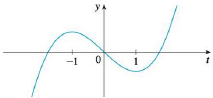Chapter 5.3, Problem 66E### Single Variable Calculus: Early Tr...

8th Edition
James Stewart
ISBN: 9781305270343

#### Solutions

Chapter
Section### Single Variable Calculus: Early Tr...

8th Edition
James Stewart
ISBN: 9781305270343
Textbook Problem

# Let F ( x ) = ∫ 1 x f ( t )   d t , where f is the function whose graph is shown. Where is F concave downward?To determine

The interval for the function F lies concave downward.

Explanation

Given information:

The interval function is F(x)=1xf(t)dt.

Here, F(x) is function of x and t is a derivative function.

Show the Theorem of fundamental calculus (part 1) as shown in below.

F(x)=axf(t)dtF(x)=f(x)

Draw the graph for f as shown in below;

Calculation:

The function F(x)=1xf(t)dt (1)

Apply the theorem of fundamentals of calculus (part 1) in Equation (1)

### Still sussing out bartleby?

Check out a sample textbook solution.

See a sample solution

#### The Solution to Your Study Problems

Bartleby provides explanations to thousands of textbook problems written by our experts, many with advanced degrees!

Get Started

#### In Problems 7-34, perform the indicated operations and simplify. 30.

Mathematical Applications for the Management, Life, and Social Sciences

#### Graph the curve x = y 2 sin y.

Single Variable Calculus: Early Transcendentals

#### Using a binomial series, the Maclaurin series for is:

Study Guide for Stewart's Multivariable Calculus, 8th